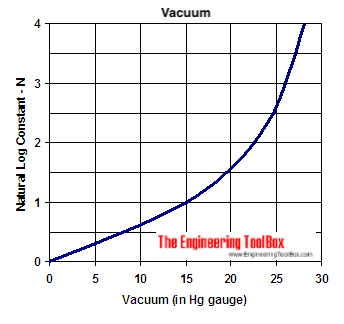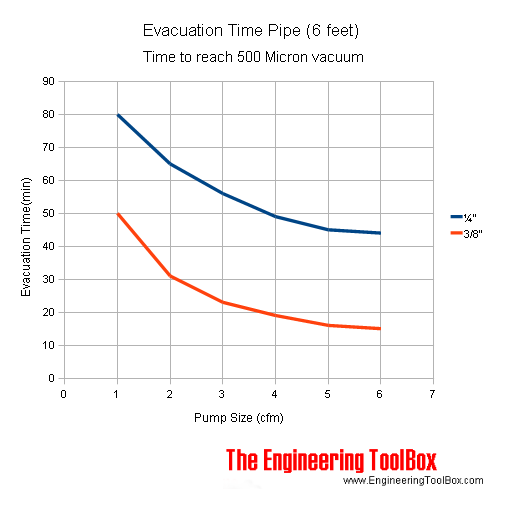Engineering ToolBox - Resources, Tools and Basic Information for Engineering and Design of Technical Applications!

# Vacuum - Evacuation Time

## Vacuum pumps - calculate flow rate and required evacuation time.

The evacuation time for a vacuum pump can be calculated as

t = V / q ln(p0 / p1)                                         (1)

where

t = evacuation time (s)

V = enclosed evacuated volume (m3, cu.ft)

q = volume flow rate capacity of the vacuum pump (m3/s, cu.ft/s)

p0 = initialization pressure - normal atmospheric pressure (mbar, mmHg)

p1 = end vacuum pressure (mbar, mmHg)

Note! Leakage through sealing's etc. should be compensated by adjusting the volume flow rate of the vacuum pump.

(1) can be simplified to

t = V / q N                                       (2)

where

N = natural log constant for the actual vacuum

N = 1 for vacuum up to 15 in Hg gauge

N = 2 for vacuum up to 22.5 in Hg gauge

N = 3 for vacuum up to 26 in Hg gauge

N = 4 for vacuum up to 28 in Hg gauge### Example - Vacuum Evacuation Time

Evacuation time of 1 m3 volume, evacuated to 500 mbar abs - vacuum pump volume flow capacity 0.1 m3/s - can be calculated as:

t = (1 m3) / (0.1 m3/s) ln((1000 mbar) / (500 mbar))

= 6.9 (s)

Evacuation to 100 mbar abs:

t = (1 m3) / (0.1 m3/s) ln((1000 mbar) / (100 mbar))

= 23 (s)

Evacuation to 10 mbar abs:

t = (1 m3) / (0.1 m3/s) ln((1000 mbar) / (10 mbar))

= 46 (s)

### Vacuum Evacuation Time Calculator

The calculator below can be used to calculate the vacuum evacuation time.

V - enclosed volume (m3, cu.ft)

q - volume flow rate capacity (m3/s, cu.ft/s)

p0 - initial pressure (mbar, mmHg)

p1 - final pressure (mbar, mmHg)

### Estimated Evacuation Time to Reach 500 Micron Vacuum - 6 feet pipe## Engineering ToolBox - SketchUp Extension - Online 3D modeling!

Add standard and customized parametric components - like flange beams, lumbers, piping, stairs and more - to your Sketchup model with the Engineering ToolBox - SketchUp Extension - enabled for use with the amazing, fun and free SketchUp Make and SketchUp Pro .Add the Engineering ToolBox extension to your SketchUp from the SketchUp Pro Sketchup Extension Warehouse!

Translate
About the Engineering ToolBox!

## Privacy

We don't collect information from our users. Only emails and answers are saved in our archive. Cookies are only used in the browser to improve user experience.

Some of our calculators and applications let you save application data to your local computer. These applications will - due to browser restrictions - send data between your browser and our server. We don't save this data.

## Citation

• Engineering ToolBox, (2005). Vacuum - Evacuation Time. [online] Available at: https://www.engineeringtoolbox.com/vacuum-evacuation-time-d_844.html [Accessed Day Mo. Year].

Modify access date.

. .

#### Scientific Online Calculator9 1